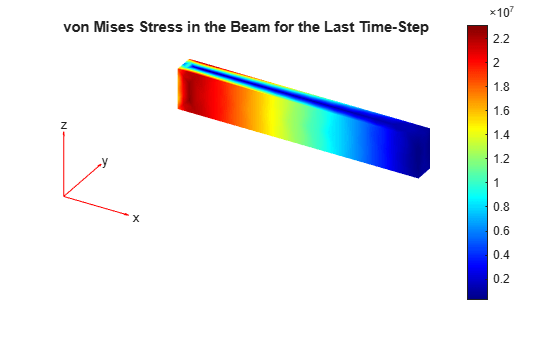# evaluateVonMisesStress

Evaluate von Mises stress for dynamic structural analysis problem

## Syntax

``vmStress = evaluateVonMisesStress(structuralresults)``

## Description

example

````vmStress = evaluateVonMisesStress(structuralresults)` evaluates von Mises stress at nodal locations for all time- or frequency-steps.```

## Examples

collapse all

Evaluate the von Mises stress in a beam under a harmonic excitation.

Create a transient dynamic model for a 3-D problem.

`structuralmodel = createpde("structural","transient-solid");`

Create the geometry and include it in the model. Plot the geometry.

```gm = multicuboid(0.06,0.005,0.01); structuralmodel.Geometry = gm; pdegplot(structuralmodel,"FaceLabels","on","FaceAlpha",0.5) view(50,20)```Specify Young's modulus, Poisson's ratio, and the mass density of the material.

```structuralProperties(structuralmodel,"YoungsModulus",210E9, ... "PoissonsRatio",0.3, ... "MassDensity",7800);```

Fix one end of the beam.

`structuralBC(structuralmodel,"Face",5,"Constraint","fixed");`

Apply a sinusoidal displacement along the y-direction on the end opposite the fixed end of the beam.

```structuralBC(structuralmodel,"Face",3, ... "YDisplacement",1E-4, ... "Frequency",50);```

Generate a mesh.

`generateMesh(structuralmodel,"Hmax",0.01);`

Specify the zero initial displacement and velocity.

`structuralIC(structuralmodel,"Displacement",[0;0;0],"Velocity",[0;0;0]);`

Solve the model.

```tlist = 0:0.002:0.2; structuralresults = solve(structuralmodel,tlist);```

Evaluate the von Mises stress in the beam.

`vmStress = evaluateVonMisesStress(structuralresults);`

Plot the von Mises stress for the last time-step.

```figure pdeplot3D(structuralmodel,"ColorMapData",vmStress(:,end)) title("von Mises Stress in the Beam for the Last Time-Step")```## Input Arguments

collapse all

Solution of a dynamic structural analysis problem, specified as a `TransientStructuralResults` or `FrequencyStructuralResults` object. Create `structuralresults` by using the `solve` function.

Example: ```structuralresults = solve(structuralmodel,tlist)```

## Output Arguments

collapse all

Von Mises Stress at the nodes, returned as a matrix. The rows of the matrix contain the values of von Mises stress at nodal locations, while the columns correspond to the time or frequency steps.

## Version History

Introduced in R2018a